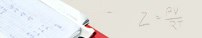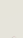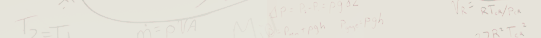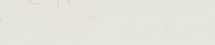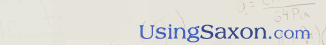Contents of the Algebra ½, 3 rd Ed (Pre-Algebra) DVD Teaching Series (123 Lessons plus 10 Supplemental Topics)Introduction: Prerequisites for the Course - How To Use The Math Book - Expectations of Students. Lesson 1: The Numbering System (Place Value, Expanded vs. Standard Notation, Reading, Writing & Adding Whole Numbers. Lesson 2: Using the Number Line to Order Numbers - Rounding Whole Numbers. Lesson 3: Patterns in Addition and Subtraction - Terminology for Subtraction. Lesson 4: Multiplication and Division - and their Patterns. Lesson 5: Word Problems requiring Addition or Subtraction. Lesson 6: Adding, Subtracting, Reading, Writing, and Rounding of Decimal Numbers. Lesson 7: Multiplying and Dividing Decimal Numbers - Estimating. Lesson 8: Multiplying and Dividing using Powers of 10's - Ordering Decimal Numbers by Their Values. Lesson 9: The Language of Geometry - (Points, Lines, Rays, Line Segments, and Angles) - Defining Perimeter. Lesson 10: Rules for Divisibility - When To Use Them. Lesson 11: Word Problems Dealing With Groups of Things - Why No Remainder! Lesson 12: Definition of Prime and Composite Numbers - Using Rules of Divisibility to find the Product of Prime Numbers. Lesson 13: Finding the Common and Greatest Common Factors (GCF). Word Problems involving Multiplication. Lesson 14: Definition of a Fraction. Expanding and Reducing Fractions. Lesson 15: Changing Fractions to Decimals and Decimals to Fractions - Repeating Decimals and How to Round Them. Lesson 16: Exponents are just Mathematical Counters. Lesson 17: Two Methods for Computing Areas of Rectangles. Lesson 18: Multiplying Whole Numbers and Fractions - Fractional Parts of Numbers - Defining using "of" when Multiplying. Lesson 19: Defining Multiplication Symbols - Multiplying and Dividing Fractions. Lesson 20: Defining Multiples and Least Common Multiple (LCM). Lesson 21: Finding the Average or "Mean." Lesson 22: Multiplying Three or More Fractions. Lesson 23: Converting Units of Length using the U.S. Standard Measuring System. Lesson 24: Converting Units of Length using the Metric System. Lesson 25: Computing Areas of Rectangles by Using the Difference of Areas. Lesson 26: Computing the Range, Mode, Median and Mean. Word Problems involving computation of the Average or "Mean." Lesson 27: The Formula for finding Areas of Triangles. Lesson 28: Converting Decimals and Mixed Numbers - "Algebraic Fractions" - Definition of an "Improper" Fraction. Lesson 29: Using/Interpreting Graphs to Depict Data. Lesson 30: Adding and Subtracting Fractions both "With and Without Equal Denominators." Lesson 31: Introduction to Mathematical Order of Operations. Lesson 32: Using Variables to Evaluate Mathematical Expressions. Lesson 33: Using Multiple Unit Multipliers by Converting Units of Area. Lesson 34: Adding Mixed Numbers - Using Fractions to Establish Rates and Ratios. Lesson 35: Subtracting using Mixed Numbers. Lesson 36: Working with Rate Word Problems. Lesson 37: Solving Basic Equations. Lesson 38: Introduction to Rectangular Coordinates. Lesson 39: Rules for Adding and Subtracting in Equations. Lesson 40: Rules for Multiplying and Dividing in Equations - Proper Use of Reciprocals. Lesson 41: Finding the Overall Average of Multiple Averages. Lesson 42: Applying Rules for using Symbols of Inclusion in Order of Operations. Lesson 43: Multiplying and Dividing using Mixed Numbers. Lesson 44: Defining Roots - Applying Rules for Use of Exponents and Roots in Order of Operations. Lesson 45: Determining Volume for Right Geometric Solids. Lesson 46: Applying Order of Operations when working with Fractions. Lesson 47: Evaluating Exponential Expressions and Radicals using Substitution. Lesson 48: Word Problems involving Fractional Parts of Numbers and Fractional Equations. Lesson 49: Computing Surface Area of Right Geometric Solids. Lesson 50: Introduction to Scientific Notation. (1.0 x 10^3) Lesson 51: Word Problems involving Decimal Parts of Numbers and Decimal Equations. Lesson 52: Applying Symbols of Inclusion to Math Problems containing Fractions. Lesson 53: Introduction to Computing Percent. Lesson 54: Using Ratios and Proportion - Evaluating Exponents and Radicals using Substitution. Lesson 55: Converting Fractions, Decimals and Percents - Applying Use of a Reference Set of Numbers. Lesson 56: Solving Equations containing Mixed Numbers. Lesson 57: Solving Word Problem Equations Containing Mixed Numbers. Lesson 58: Introduction to Basic Distance Word Problems. Lesson 59: More Ratio and Proportion Problems using Fractions. Lesson 60: Formulas For Working with Circles. Lesson 61: More Simple Equations. Lesson 62: Word Problems using Fractions. Lesson 63: Calculating Changing Rates by Establishing an Initial Rate. Lesson 64: Formulas for Working with Semi-Circles. Lesson 65: Proportions of Mixed Numbers - Applying Proportions to Similar Triangles. Lesson 66: Word Problems using Ratios and Proportions. Lesson 67: Using Ratios to Compare Different Sizes, Shapes, Weights, etc. Lesson 68: Word Problems Involving Percents less than 100. Lesson 69: Adding Signed Numbers by Using their Absolute Values. Lesson 70: Rules Governing the Addition of Signed Numbers. Lesson 71: Applying the Rules for Powers and Roots to Fractions. Lesson 72: Graphing "Inequalities." Lesson 73: Determining Formulas for Volumes of Right Circular Cylinders. Lesson 74: Using Parenthesis with Signed Numbers. Lesson 75: Applying Advanced Techniques to Solve Word Problems involving Ratios. Lesson 76: Using Scientific Notation to Multiply Large Numbers. Lesson 77: Word Problems using Percents Greater than 100. Lesson 78: Application of the "Double Negative." Lesson 79: Simplifying Notations using Multiple Parenthesis or Brackets. Lesson 80: Word Problems using "Increases" in Percents. Lesson 81: Rules for the Multiplication and Division of Signed Numbers. Lesson 82: Evaluating Mathematical Expressions containing Signed Numbers. Lesson 83: Word Problems Involving Use of Proportions. Lesson 84: Using Negative Coefficients - Properties of Equality. Lesson 85: Equations of a Line - Procedures for Graphing a Straight Line. Lesson 86: The "Words of Mathematics." - Applying the Meaning of Certain Words to solving Algebraic Equations. Lesson 87: The Properties of Algebra. Lesson 88: Finding the Surface Area of a Right Geometric Solid. Lesson 89: The definition of "Trichotomy" and the proper use of Symbols of Negation. Lesson 90: The meaning of Algebraic Phrases or Sentences. Lesson 91: Using Order of Operations and Symbols of Inclusion with Signed Numbers. Lesson 92: Estimating the Roots of Numbers. (Neat Formula in APPENDIX E) Lesson 93: Working with Symbols of Inclusion in Fractions with Extended Numerators & Denominators. Lesson 94: Introduction to Adding Like Terms - Definition of a "Term." Lesson 95: Solving Equations with Variables on Both Sides. Lesson 96: Solving Equations with Multiple Terms. Lesson 97: Solving More Complicated Equations. Lesson 98: Defining Adjacent, Complementary, and Supplementary Angles - How to Measure Angles. Lesson 99: Using Exponents and Symbols of Inclusion with both Positive and Negative Bases. Lesson 100: More Advanced Ratio Problems. Lesson 101: Multiplying Exponential Expressions using Bases containing Numbers or Variables. Lesson 102: Adding More Advanced Terms. Lesson 103: Use of the Distributive Property with Variables. Lesson 104: Classifying Triangles by using their Sides and Angles. Lesson 105: Evaluating the powers of more Complicated Terms with Negative Bases. Lesson 106: Roots of Negative numbers - Working with Negative Exponents - Effects of a Zero Exponent. Lesson 107: Using Roman Numerals - Why? Lesson 108: Percents as a Fraction. Lesson 109: Calculating Simple and Compound Interest. Lesson 110: Understanding Markup and Markdown in the Business World. Lesson 111: Calculating Commission and Profit. Lesson 112: Introduction to Probability. Lesson 113: Using a Ruler marked with Metric and U.S. Measurements. Lesson 114: Probability with Dependent and Independent Events. Lesson 115: Definition and Description of Polygons, and Congruence and Transformations. Lesson 116: Calculating Areas of Trapezoids and Parallelograms. Lesson 117: Pythagorean Theorem - Proof of the Pythagorean Theorem - Effects of x^2. Lesson 118: Volume Conversions using U.S. Measurements. Lesson 119: Volume Conversions using Metric Measurements. Lesson 120: Calculating the Volume of Pyramids, Cones and Spheres, and the Surface Area of Pyramids and Cones. Lesson 121: Visualizing the Formation of Solids - Applying the Rules of Symmetry. Lesson 122: Introduction to Permutations. Lesson 123: The Subsets of Real Numbers - Difference between Numbers and Numerals. NOTE: In this book, there are an additional 10 Supplemental Topics that are described within the appropriate lessons of the course telling the student when to review each appendix related to that particular lesson. APPENDIX A: Geometric Constructions. APPENDIX B: Using Stem and Leaf Plots. APPENDIX C: Understanding Base 2 in Comparisn with Base 10. APPENDIC D: Working with Angles and Arcs. APPENDIX E: Formula for Approximating Roots. APPENDIX F: Polynomials. APPENDIX G: Using Transformational Geometry. APPENDIX H: Graphing Parabolas. APPENDIX I: Calculating the "Slope" of a line. APPENDIX J: Introduction to Basic Trigonometry. Home | Review the book | Purchase book | Testimonials | Newsletter | Contact Us © 2006 - 2019 AJ Publishers, LLC